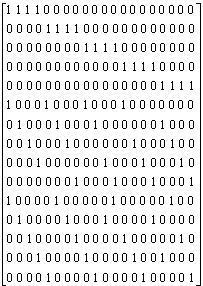## Wednesday, 18 July 2007

### The Inspiration Of Ldpc Codes

The full name of LDPC code is low-density parity-check code, which means only few 1’s are shown on the parity-check matrix. For a linear block code, such as LDPC codes, we can find a fundamental decoding condition, cHT=0 where c is a code word and H is a parity-check matrix. If we do the matrix multiplication, we may get the following results. For example, c0+c1+c3=0; c3+c4+c7=0 where ci is the ith coded bit. Therefore, c0 is only influenced by c1 and c3.

Gallager proposed LDPC codes in 1962. The inspiration of LDPC codes is from less influence among different coded bits. For any code word, every coded bit can be corrected by two other bits assumed that there are three 1’s in a parity-check matrix row. If intrinsic information (received probability) doesn’t affect every coded bit, we can correct error bits through other extrinsic information (propagated probability). As block size increases, the coded bits become less correlated. Thus, we think of every coded bit as an independent bit and do an iterative algorithm called belief propagation. Finally, the decoded bits will be determined by both intrinsic information and extrinsic information.

http://www.shvoong.com/exact-sciences/engineering/electrical-engineering/communications-signal-processing/1630485-inspiration-ldpc-codes/

## Sunday, 8 July 2007

### Properties of LDPC Codes

1.Properties of LDPC Codes
1.1 Sparse Parity Matrix
A sparse matrix is a matrix populated with few ones in each row and column. More precisely, the ratio of nonzero entries into that matrix is kept low. This is the reason why they are called “low-density”. In particular, an (n,wc,wr) low-density-code is a code of block length n with a matrix like that of Fig..1 whose parity matrix has wc 1’s in each column, and wr 1’s in each row.Fig. 1 low-density code matrix; N=20, wc =3, wr =4

1.2 Regular LDPC Codes
A regular LDPC code is a linear block code whose parity matrix H contains exactly wc 1’s in each column and wr = wc(n/n-k) 1’s in each row. The code rate is k/n and wc is extremely smaller than (n-k). Similarly, wr is extremely smaller than n. In , Markey shows that wc = 3 is necessary for good codes.

1.3 Irregular LDPC Codes
If the number of 1's per column or row is not constant, the code is an irregular LDPC code. It’s very easy to determine whether an LDPC code is regular or irregular through its graphical representation. Usually, irregular LDPC codes outperform regular LDPC codes.

2. Characteristics of LDPC Codes
In some specific situations, we can get the following characteristics.
2.1 Large dmin
Some facts can help LDPC codes achieve this goal. First, any two columns have an overlap of at most one 1. Secondly, the sparse property allows us to avoid over-lapping. Less over-lapping means high independence among different coded bits. The condition makes LDPC decoder good decoding ability and low bit error rate.

2.2 Low Complexity
The "Low Density" characteristic only applies to the H matrix (i.e., the parity check matrix). H matrix is a decision criterion and represents decoding algorithm. Thus, lower density in the H matrix yields low-complexity in the decoder.